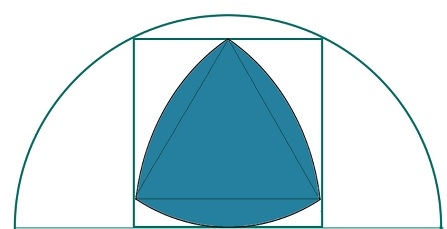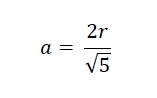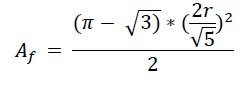# Biggest Reuleaux Triangle inscirbed within a square inscribed in a semicircle?

Here we will see the area of biggest Reuleax triangle inscribed within a square which is inscribed in a semicircle. Suppose the radius of the semicircle is R, are side of the square is ‘a’ and the height of the Reuleax triangle is h.We know that the side of the square inscribed in a semicircle is −The height of the Reuleaux triangle is the same as a. So a = h. So the area of the Reuleaux triangle is −## Example

#include <iostream>
#include <cmath>
using namespace std;
float areaReuleaux(float r) { //radius of the semicircle is r
if (r < 0) //if r is negative it is invalid
return -1;
float area = ((3.1415 - sqrt(3)) * (2*r/(sqrt(5))) * (2*r/(sqrt(5))))/2;
return area;
}
int main() {
}
Area of Reuleaux Triangle: 36.0819